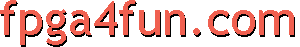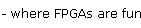## FPGA software 3 - FPGA simulation

Once the hardware design entry is completed (using either a schematic or an HDL), you may want to simulate your design on a computer to gain confidence that it works correctly before running it in an FPGA. Simulation requires a form of input stimulus and then FPGA simulator software can determine the corresponding outputs.

There are two ways to create input stimulus:

• Using an interactive waveform editor (easy).
• Using a testbench (a bit harder).

#### Interactive waveform editor

Using an interactive waveform editor, you enter the shape of the inputs (with a few clicks of your computer mouse), and the simulator software draws the shape of the outputs.

Interactive waveform editors were nice for beginners (easy to learn) but are now a dying breed (as much for their limitations than marketing reasons) so you will probably have to do it the hard way...

#### Testbench

A testbench is a non-synthesizable HDL design that creates stimulus for another (usually synthesizable) design. For example, let's assume that this synthesizable "gates" circuit needs to be exercised.

 module gates(a, b, q, r); input a, b; output q, r; assign q = a & b;    // one AND gate assign r = a | b;     // one OR gate endmodule

A testbench could be:

 module testbench_for_gates; // we create some stimulus by toggling the signals "a" and "b" every 50 time-units reg a, b; initial begin   a = 0; b = 0;   #50 a = 1;   #50 a = 0; b = 1;   #50 a = 1;   #50 \$finish; end // and we apply the stimulus to "gates". In response, "gates" drives "q" and "r" signals wire q, r; gates my_gates(a, b, q, r); // and we can verify that "q" and "r" have the right values always @(a or b) if(q != (a & b)) \$display("ERROR in signal q"); always @(a or b) if(r != (a | b)) \$display("ERROR in signal r"); endmodule

The testbench is usually written in the same language (VHDL or Verilog) than your circuit under test.

FPGA software provide HDL simulators, like ISim and ModelSim. Or check the List of Verilog simulators from Wikipedia.